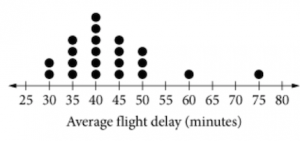# 4 SAT Math Questions of the Day That Might Catch You by Surprise

#### 1. Equation of a Circle (October 1st, 2015)

A circle in the $$xy$$-plane has its center at $$(-100, 221)$$ and a diameter of 17. Which of the following is an equation of the circle?

A) $$(x + 100)^2 + (y - 221)^2 = 72.25$$
B) $$(x + 100)^2 + (y - 221)^2 = 289$$
C) $$(x - 100)^2 + (y + 221)^2 = 72.25$$
D) $$(x - 100)^2 + (y + 221)^2 = 289$$

In the $$xy$$-plane, the equation of a circle with center $$(h, k)$$ and radius $$r$$ is given by $$(x - h)^2 + (y - k)^2 = r^2$$. Since the diameter is 17, the radius is 8.5. Therefore, the equation of the circle is

$(x + 100)^2 + (y - 221)^2 = 8.5^2$ $(x + 100)^2 + (y - 221)^2 = 72.25$

#### 2. Dotplots (October 9th, 2015)For each of the largest 21 airline companies in Europe, the average flight delay was calculated and is shown in the dot plot above. If the dots representing the two airlines with the longest average flight delays are removed from the dot plot, what will happen to the mean, median, and range of the new data set?

A) Only the mean will decrease.
B) Only the mean and range will decrease.
C) Only the mean and median will decrease.
D) The mean, median, and range will decrease.

To read a dotplot, simply count the number of dots above the axis. For example, there are 2 airlines with an average flight delay of 30 minutes. As you can see, there are a total of 21 dots to represent the 21 airline companies that were studied.

Getting back to the question, it's obvious that the mean will decrease because we're removing the data points with the two highest values, the ones that now bring up the average the most. The range will decrease because the difference between the minimum and the maximum values will decrease (the new maximum will be 50 instead of 75).

Currently, the median is represented by 11th airline, which has an average flight delay of 40 minutes. After the two airlines are removed, the median will be represented by the 10th airline, which also has an average flight delay of 40 minutes. Therefore, the median will not change. Only the mean and the range will decrease.

#### 3. Conditional Probability (November 10th, 2015)

Laptop Use on Vacation

 Male Female Total Brought a laptop 112 212 324 Did not bring a laptop 355 321 676 Total 467 533 1,000

The table above gives the results of a survey about whether or not participants brought a laptop on their most recent vacation. If one of the participants is selected at random, what is the probability that the participant is female, given that the participant brought a laptop on vacation? (Round your answer to the nearest hundredth.)

A) .21
B) .32
C) .40
D) .65

Conditional probability means that we're not choosing from the entire group. The people we're choosing from must meet a condition, or requirement. In this case, we're only choosing from the people who brought a laptop on vacation. That given knowledge about the participant narrows the selection group down to 324 people. Of those 324 people, 212 were female. Therefore, the probability that a female is chosen from the participants who brought a laptop on vacation is

$\dfrac{212}{324} = 0.65$

#### 4. Radians (December 2nd, 2015)

If $$\theta = 330^\circ$$, what is the value of $$\theta$$ in radians?

A) $$\dfrac{3\pi}{2}$$

B) $$\dfrac{5\pi}{3}$$

C) $$\dfrac{7\pi}{4}$$

D) $$\dfrac{11\pi}{6}$$

Radians, just like degrees, are units used to measure angles, in the same way centimeters, just like inches, are units used to measure distance. The conversion is $$180^\circ = \pi \text{ radians}$$. Don't be confused by the $$\pi$$. The equation is just another conversion factor, just like $$1 \text{ inch} = 2.54 \text{ centimeters}$$.

Using the conversion factor,

$330^\circ \times \dfrac{\pi \text{ radians}}{180^\circ} = \dfrac{11\pi}{6}$